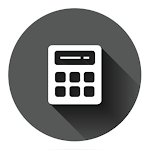ID: club.onepercent.calculator

• Author:

• Version:

1.1.6

• Update on:

## The description of Calculator+ - Unit Conversion

Calculator+ is an advanced alternative to the Built-In Calculator in Android.
It allows you to easily handle all the calculations of everyday life, be it simple mathematical equations, or complex scientific equations. It's an app with a sleek, interactive, and customizable graphical user interface.

Calculator+ is
- Free with no hidden charges!
- Available for all platforms!

Features:

1. General Calculator
- A Calculator which enables you to do simple and advanced calculations with ease.
- Display the result either in Standard form or Scientific form based on your requirements.
- Have the freedom to choose the precision of your answers for accuracy depending on the purpose of the calculation.
- Log all previous calculations.

2. Percentage Calculator
- Perform Percentage based calculations with ease without having to remember formulae.

3. BMI Calculator
- Calculate a person's BMI (Body Mass Index) with the touch of a button.

4. Investment Calculator
- Calculate the Return on your Investment with the interest rate being yearly, half-yearly, quarterly, monthly, or daily.

5. EMI and Loan Calculator
- Calculate your EMI and the total Interest payable.

6. Tax (VAT / GST) Calculator
- Get the total price by entering the original price and the tax rate.

7. Length Conversion
- Convert between Length units supporting all major Length Units such as Kilometer, Meter, Decimeter, Centimeter, Millimeter, Micrometer, Nanometer, Picometer, Nautical Mile, Mile, Yard, Foot, Inch, Lunar Distance, Astronomical Unit, Light Year.

8. Area Conversion
- Convert between Area units supporting all major Area Units such as Square Kilometer, Square Meter, Hectare, Square Decimeter, Square Centimeter, Square Millimeter, Square Micron, Acre, Square Mile, Square Yard, Square Yard, Square Foot, Square Inch, Square Rod.

9. Volume Conversion
- Convert between Volume units supporting all major Volume Units such as Liter, Hectoliter, Deciliter, Centiliter, Milliliter, Cubic Meter, Cubic Decimeter, Cubic Centimeter, Cubic Millimeter, Cubic Foot, Cubic Inch, Cubic Yard, Acre Foot.

10. Temperature Conversion
- Convert between Temperature units supporting all major Temperature Units such as Celsius, Fahrenheit, Kelvin, Rankine, and Réamur.

11. Speed Conversion
- Convert between Speed units supporting all major Speed Units such as Lightspeed, Meter per second, Kilometer per second, Mile per hour, Kilometer per hour, Knot, Foot per second, and Inch per second.

13. Time Conversion
- Convert between Time units supporting all major Time Units such as Century, Decade, Year, Week, Day, Hour, Minute, Second, Millisecond, Microsecond, and Picosecond.

14. Mass Conversion
- Convert between Mass units supporting all major Mass Units such as Kilogram, Gram, Pounds, Tonne, Milligram, Microgram, Quintal, Ounce, Carat, and Grain.

15. Numeral Conversion
- Convert between Numeral units supporting all major Numeral Units such as Binary, Octal, Decimal, and Hexadecimal.

16. Quantity Conversion
- Convert between Quantity units supporting all major Quantity Units such as Liter, Gallon, Milliliter, Fluid Ounce, Cup, TableSpoon, TeaSpoon, Quart, and Pint.

17. Age Calculator
- Know your exact age with a report on how many days and months are remaining before your next birthday.

18. Theme Customization
- Customize the whole app. Change the theme of the app to your liking.

This app is brought to you by the company OnePercent, based in Bangalore, India.

Website: www.OnePercent.club

Social Media